# JavaScript Data Types

## JavaScript Data Types with Examples

In this article, I am going to discuss JavaScript Data Types with Examples. Please read our previous article where we discussed the JavaScript Comments and Statements. At the end of this article, you will understand the following pointers in detail.

1. What is a Data Type?
2. How to define a variable in JavaScript?
3. How to Check the data type of a variable in JavaScript?
4. Data types in JavaScript are Dynamic
5. Types of Data Types in JavaScript
6. What is an undefined data type in JavaScript?
7. What is the difference between null and undefined in JavaScript?
8. Primitive and Reference Value in JavaScript
##### How to define a variable in JavaScript?

To define a variable in JavaScript, we need to use the var keyword as shown below. While defining a variable we need to provide the variable name and value that is going to store in the variable.

var x = 10;

In JavaScript, the data types are defined by using the inferring process. Inferred means the variable (in our example x) is going to be a number data type or string data type or Boolean data type is defined by the value assigned to it.

Note: If you want to see the data type of a variable then you can use typeof function in JavaScript.

##### Example: Checking the data type of a Variable in JavaScript

Please have a look at the below example. Here, we define the x variable and assigned the value 10 to it. And we are logging the data type in the console window using the console.log method. As discussed, the typeof method gives the data type.

```<html>
<title>JavaScript DataType example</title>
<body>
<script>
var x = 10;
console.log("x data type : " + typeof(x));
</script>
</body>
</html>
```

Once you run the above program, open the browser console window by pressing the F12 key or Function + F12 key and you will get the following output. As we are assigning a number (i.e. 10) to the variable x, so it is showing the data type as a number.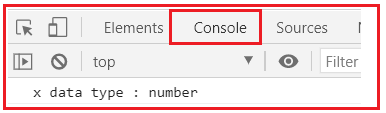Note: The point that you need to remember is, depending open the value assigned to the variable the data type is defined in JavaScript.

##### Data types in JavaScript are Dynamic

JavaScript data types are converted automatically as needed during the script execution. In other words, we can say that the same variable can be used to hold different data types. Let us understand what do you mean by JavaScript Data Types are Dynamic. For this, please have a look at the following example.

If you see, to the variable x, first we assign the value 10, at this point of time, the data type is number. Then we assign a string value (javascript) to the same variable x, so the data type at this point of time is a string. Again, we assign a Boolean value (true) to the same variable x and hence the data type also changed to Boolean.

```<html>
<title>JavaScript Data Type Example</title>
<body>
<script>
var x = 10;
console.log("x data type : " + typeof(x));  //number
x = "javasript";
console.log("x data type : " + typeof(x)); //number
x = true;
console.log("x data type : " + typeof(x)); //boolean
</script>
</body>
</html>
```

When you run the above code, you will get the following output in the browser console. You can get the browser console by pressing the F12 or function F12 key.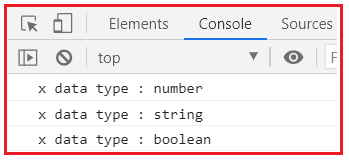Note: The JavaScript data types are dynamic, which means based on the value assigned to it, the data types are defined. So, JavaScript is a dynamic language.

##### Types of Data Types in JavaScript:

There are three primary types of Data Types in JavaScript. They are Number, String, and Boolean.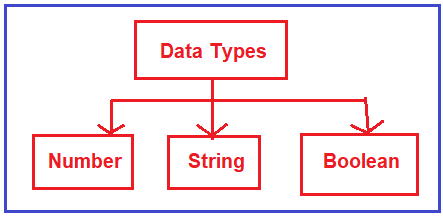In JavaScript, we don’t differentiate between normal numbers, decimal, double, etc. like we have an integer, double, decimal in other programming languages such as C#, Java. In JavaScript, it is just a number. But if you want to figure out whether it is a normal number or decimal, then you need to write some logic in JavaScript and that we will see in our upcoming articles.

##### What is an undefined data type in JavaScript?

If you declare a variable without assigning any value to it, then the data type of that variable becomes undefined. Let us understand this with an example. In the below example, we declare the variable x without assigning any value and then we try to figure the data type of the variable x using the typeof function.

```<html>
<title>JavaScript Undefined Example</title>
<body>
<script>
"use strict"
var x;
console.log("x Data Type: " + typeof(x));
</script>
</body>
</html>
```

Output: When you run the above code, you should get the data type as undefined in the browser console window as shown in the below image.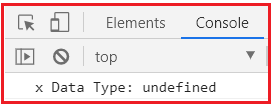So, undefined is a state in JavaScript where the variable is declared but is not assigned.

##### What is the difference between null and undefined in JavaScript?

Don’t confuse undefined with null. Both are two different things altogether. Null represents nothing. That means null is neither a number, a string, or a Boolean. Null means nothing. Sometimes, in your program you want to represent nothing then at that time you need to use null. On the other hand, if you create a variable without assigning any value, then the data type of that variable is undefined.

Let us understand the above two things with an example. In the below example, we declare the variable x without assigning any values and hence it will print undefined. On the other hand, we declare the variable y and assign this variable with the value null and when you print this variable, you will get null.

```<html>
<title>JavaScript Undefined Example</title>
<body>
<script>
"use strict"
var x;
console.log("x : " + x);
var y = null;
console.log("y : " + y);
</script>
</body>
</html>
```

Output: When you run the above code, you will get the following output in the browser console window.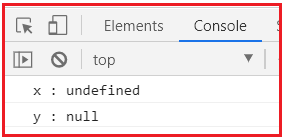##### Primitive and Reference Value in JavaScript:
###### Primitive Values:

Primitive values are the simple piece of data that are stored on the stack. That means the value is stored directly in the location from where the variable is accessed. The JavaScript primitive types are Undefined, Null, Boolean, Number, or String. Many languages consider string as a reference type and not a primitive type but JavaScript considers it a primitive type.

###### Reference Values:

Reference values are objects that are stored in heap. That means the value stored in the variable location as a pointer to a location in memory where the object is stored. In JavaScript, there are 3 primary data types, 2 composites (reference) data types, and 2 special data types.

1. String
2. Number
3. Boolean
1. Undefined
2. Null
1. Object
2. Array
##### String Data Type in JavaScript:

It represents a sequence of characters enclosed with single quotes (‘) and double quotes (‘’) respectively.
Example:
var country=”India”;
var day=’Monday’;

##### Number Data Type:

The number of data types deal with digits. It covers both floating-point numbers as well as integers. Floating-point numbers are like 4.22 and 0.98. Integer numbers are like 25, 125, and 1200.
Example:
var age=23;

##### Infinity and NAN:

Infinity is a property of a number.it represents mathematical infinity. Example: var infinity=1e400*1250;

NaN stands for not a number and it’s a result of a mathematical operation that doesn’t have sense. This popup when we divide 0 by 0. Example: var x=0/0;

##### Boolean Data Type in JavaScript:

The Boolean type has two values, true and false.
Example:
var exist= true;
var profit=false;

##### Undefined Data Type in JavaScript:

Undefined has only one value Undefined. It means a variable has not been defined, no such variable exists in the current scope. In simple words, nothing is stored in the variable. Undefined is different from that null.
Example:
var x;
In the above variable name x, no value is assigned to it.

##### Null Data Type in JavaScript:

The null type has only one value Null. It means that an object exists and is empty. In simple words, the null values mean no data or this variable doesn’t have any data stored in it.
Example:
Var x=null;

##### Object Data Type:

Everything is an object in JavaScript. JavaScript is an object-based language.
Example: var objectname=new Object();

##### Array Data Type:

The array is an ordered sequence of elements. If we want multiple variables of the same or different types then remembering the names of variables is a problem. The concept of the array allows us to store different data type data information under the same name in a sequence ordered way. It helps in creating many variables. There is no need for remembering their names because they all have the same name but different positions in the array. The counting in an array starts from 0, not from 1, which means the first location is the 0th location and the 12th is the 11th.

Example: var b=new Array (“one”,”two”,”three”,”four”);
In the above example, we have declared an array variable.

In the next article, I am going to discuss JavaScript Variables with examples. Here, in this article, I try to explain JavaScript Data Types with Examples. I hope you enjoy this JavaScript Data Types with Examples article.